# Lamé coefficients

(diff) ← Older revision | Latest revision (diff) | Newer revision → (diff)

of an orthogonal curvilinear coordinate system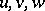in space

The quantities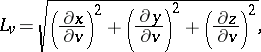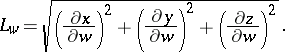The Lamé coefficients in the plane are defined similarly. In terms of the Lamé coefficients in the coordinatesone can express the element of arc length: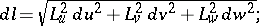the element of surface area: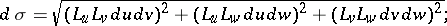and the volume element:The Lamé coefficients occur in the expressions for the operations of vector analysis in the coordinates: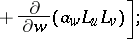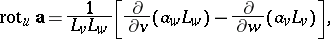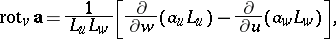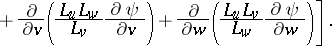For the Lamé coefficients of various orthogonal curvilinear coordinates see the corresponding articles on those coordinates.

Lamé coefficients were introduced by G. Lamé .

How to Cite This Entry:
Lamé coefficients. Encyclopedia of Mathematics. URL: http://encyclopediaofmath.org/index.php?title=Lam%C3%A9_coefficients&oldid=13659
This article was adapted from an original article by V.I. Bityutskov (originator), which appeared in Encyclopedia of Mathematics - ISBN 1402006098. See original article